Next: SLA_UE2PV - Pos/Vel from Universal Elements
Up: SUBPROGRAM SPECIFICATIONS
Previous: SLA_TPV2C - Plate centre from and x,y,z

## SLA_UE2EL - Universal to Conventional Elements

ACTION:
Transform universal elements into conventional heliocentric osculating elements.

CALL:
CALL sla_UE2EL ( U, JFORMR, JFORM, EPOCH, ORBINC, ANODE, PERIH, AORQ, E, AORL, DM, JSTAT)

GIVEN:

 D(13) U universal orbital elements (updated; Note 1) (1) combined mass (M+m) (2) total energy of the orbit (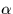) (3) reference (osculating) epoch (t0) (4-6) position at reference epoch (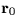) (7-9) velocity at reference epoch (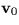) (10) heliocentric distance at reference epoch (11)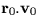(12) date (t) (13) universal eccentric anomaly (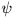) of date, approx JFORMR I requested element set (1-3; Note 3)

RETURNED:

 I JFORM element set actually returned (1-3; Note 4) EPOCH D epoch of elements (t0 or T, TT MJD) ORBINC D inclination (i, radians) ANODE D longitude of the ascending node (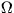, radians) PERIH D longitude or argument of perihelion (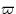or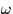, radians) AORQ D mean distance or perihelion distance (a or q, AU) E D eccentricity (e) AORL D mean anomaly or longitude (M or L, radians, JFORM=1,2 only) DM D daily motion (n, radians, JFORM=1 only) JSTAT I status: 0 = OK -1 = illegal PMASS -2 = illegal JFORMR -3 = position/velocity out of allowed range

NOTES:
1.
The universal'' elements are those which define the orbit for the purposes of the method of universal variables (see reference 2). They consist of the combined mass of the two bodies, an epoch, and the position and velocity vectors (arbitrary reference frame) at that epoch. The parameter set used here includes also various quantities that can, in fact, be derived from the other information. This approach is taken to avoiding unnecessary computation and loss of accuracy. The supplementary quantities are (i), which is proportional to the total energy of the orbit, (ii) the heliocentric distance at epoch, (iii) the outwards component of the velocity at the given epoch, (iv) an estimate of, the universal eccentric anomaly'' at a given date and (v) that date.
2.
The universal elements are with respect to the mean equator and equinox of epoch J2000. The orbital elements produced are with respect to the J2000 ecliptic and mean equinox.
3.
Three different element-format options are supported, as follows.

JFORM=1, suitable for the major planets:


EPOCH 		 = 		 epoch of elements t0 (TT MJD)
ANODE 		 = 		 longitude of the ascending node(radians)
PERIH 		 = 		 longitude of perihelion(radians)
AORQ 		 = 		 mean distance a (AU)
E 		 = 		 eccentricity e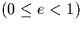AORL 		 = 		 mean longitude L (radians)
DM 		 = 		 daily motion n (radians)


JFORM=2, suitable for minor planets:


EPOCH 		 = 		 epoch of elements t0 (TT MJD)
ANODE 		 = 		 longitude of the ascending node(radians)
PERIH 		 = 		 argument of perihelion(radians)
AORQ 		 = 		 mean distance a (AU)
E 		 = 		 eccentricity eAORL 		 = 		 mean anomaly M (radians)


JFORM=3, suitable for comets:


EPOCH 		 = 		 epoch of perihelion T (TT MJD)
ANODE 		 = 		 longitude of the ascending node(radians)
PERIH 		 = 		 argument of perihelion(radians)
AORQ 		 = 		 perihelion distance q (AU)
E 		 = 		 eccentricity e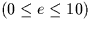4.
It may not be possible to generate elements in the form requested through JFORMR. The caller is notified of the form of elements actually returned by means of the JFORM argument:


JFORMR 		 JFORM 		 meaning

1 		      1 		 OK: elements are in the requested format
1 		      2 		 never happens
1 		      3 		 orbit not elliptical
2 		      1 		 never happens
2 		      2 		 OK: elements are in the requested format
2 		      3 		 orbit not elliptical
3 		      1 		 never happens
3 		      2 		 never happens
3 		      3 		 OK: elements are in the requested format

5.
The arguments returned for each value of JFORM (cf Note 5: JFORM may not be the same as JFORMR) are as follows:


JFORM 		 1 		 2 		 3

EPOCH 		 t0 t0 T
ORBINC 		 i i i
ANODEPERIHAORQ 		 a a q
E 		 e e e
AORL 		 L M -
DM 		 n - 		 -


where:


t0 is the epoch of the elements (MJD, TT)
T is the epoch of perihelion (MJD, TT)is the longitude of the ascending node (radians)is the longitude of perihelion (radians)is the argument of perihelion (radians)
a is the mean distance (AU)
q is the perihelion distance (AU)
e is the eccentricity
L is the longitude (radians,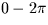)		 M is the mean anomaly (radians,)		 n is the daily motion (radians)
- 		 means no value is set

6.
At very small inclinations, the longitude of the ascending node ANODE becomes indeterminate and under some circumstances may be set arbitrarily to zero. Similarly, if the orbit is close to circular, the true anomaly becomes indeterminate and under some circumstances may be set arbitrarily to zero. In such cases, the other elements are automatically adjusted to compensate, and so the elements remain a valid description of the orbit.

REFERENCES:
1.
Sterne, Theodore E., An Introduction to Celestial Mechanics, Interscience Publishers, 1960. Section 6.7, p199.
2.
Everhart, E. & Pitkin, E.T., Am. J. Phys. 51, 712, 1983.Next: SLA_UE2PV - Pos/Vel from Universal Elements
Up: SUBPROGRAM SPECIFICATIONS
Previous: SLA_TPV2C - Plate centre from and x,y,z

SLALIB --- Positional Astronomy Library